### Choose language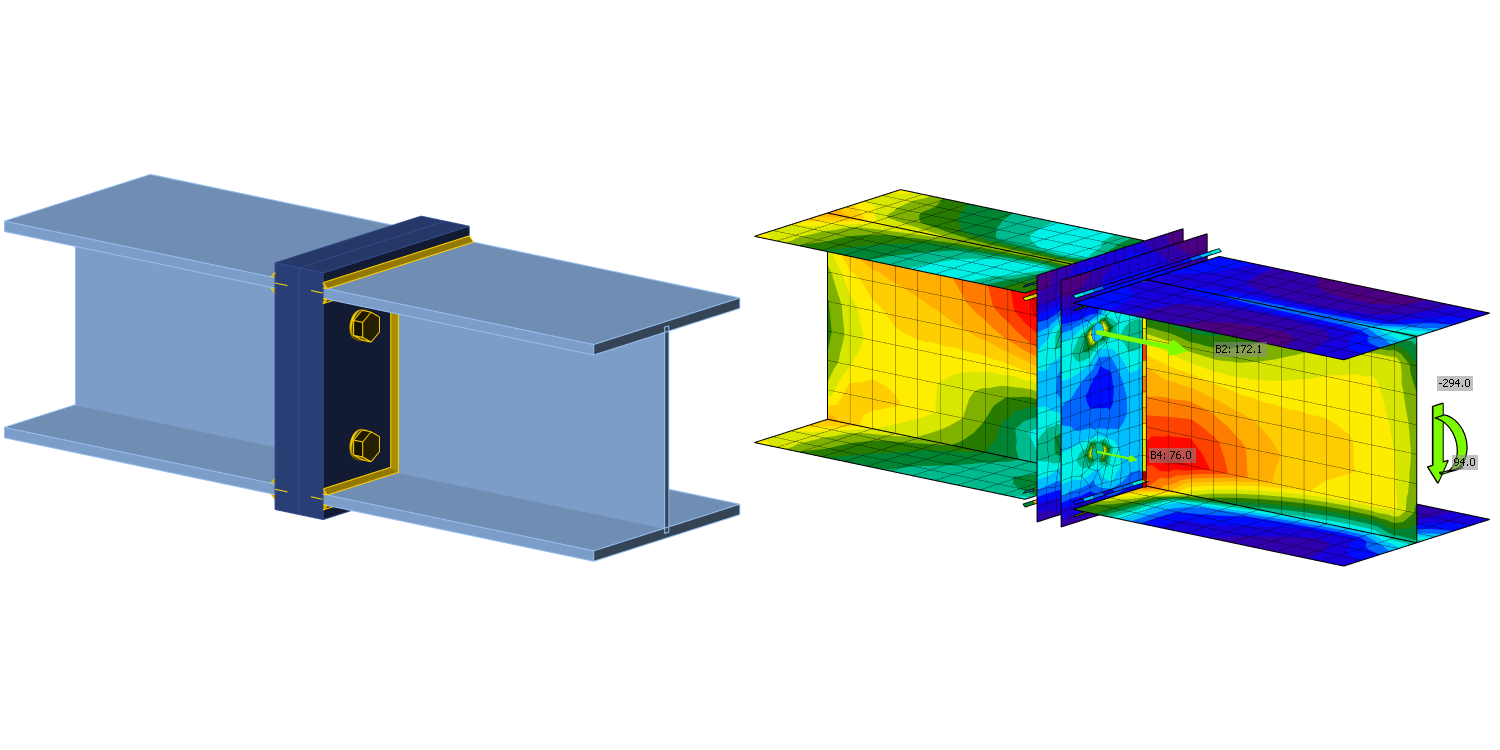# Bolted connection - Interaction of shear and tension

This is a selected chapter from book Component-based finite element design of steel connections by prof. Wald et al. The chapter is focused on verification of bolts loaded by a combination of tension and shear.
$$DescriptionThe objective of this chapter is a verification of the component-based finite element method (CBFEM) for the interaction of shear and tension in a bolt to an analytical model (AM). A beam-to-beam joint with end plates and two rows of bolts was selected for verification; see Fig. 5.5.1. The bending stiffness of the joint is high enough to be classified as rigid.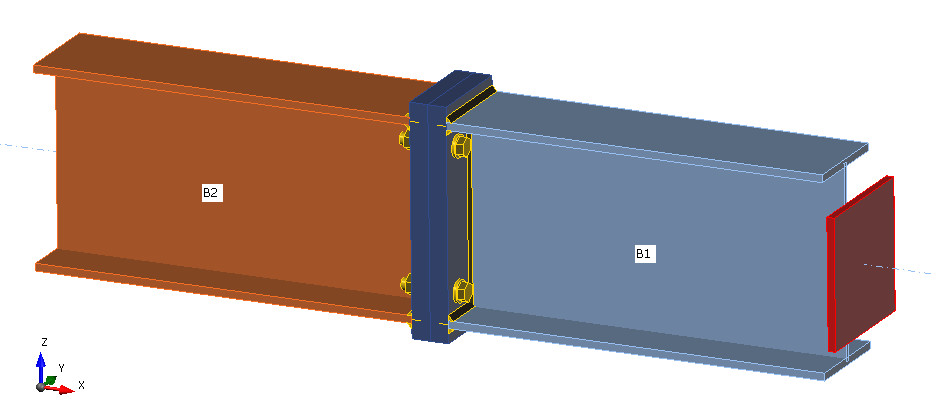Fig. 5.5.1 Joint arrangement of bolted beam-to-beam jointAnalytical modelBolt resistance in interaction of shear and tension is designed according to Tab. 3.4 in chapter 3.6.1 in EN 1993-1-8:2005. A bilinear relation is used. The geometry and the end plate dimensions of the joint are selected to limit the design resistance of the joint by bolt failure. The design resistance of equivalent T-stub in tension is modeled according to Tab. 6.2 in chapter 6.2.4 in EN 1993‑1‑8:2005.Verification of resistanceParameters of the model are a bolt diameter and a beam dimension; see Figs 5.5.2 to 5.5.5. Dimensions of the end plate and the bolt distances are modified to limit the joint resistance by the bolt failure. The shear and bending resistance of the joint is compared in loading at the bolt failure. The results are summarised in Tab. 5.5.1 and 5.5.2.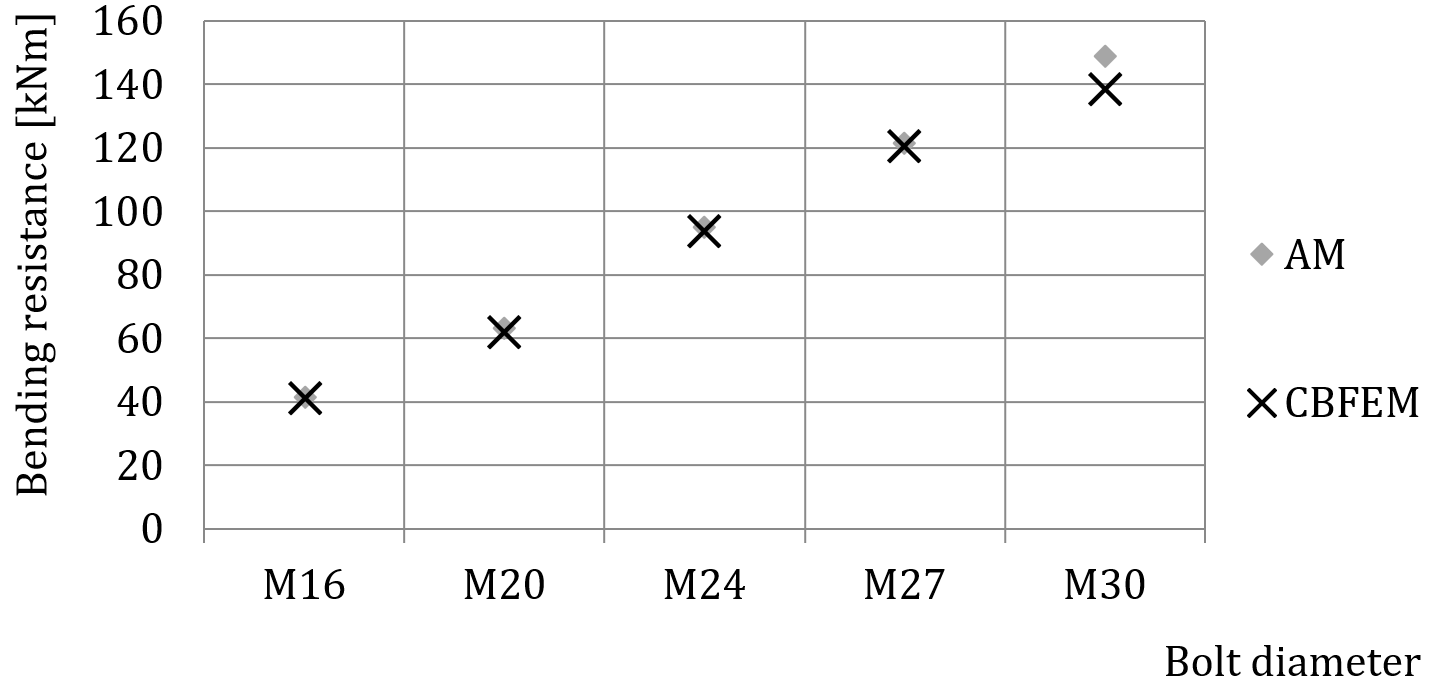Fig. 5.5.2 Sensitivity study for resistance in bending with variation of bolt diameter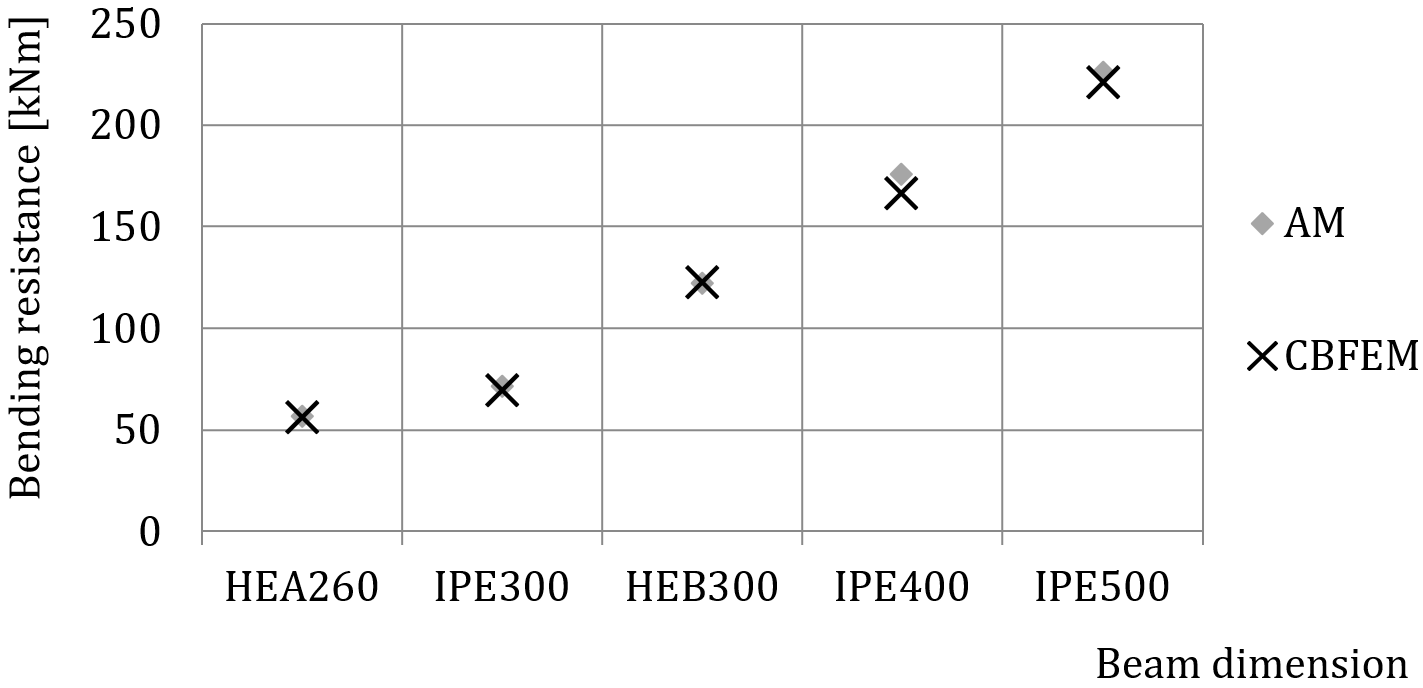Fig. 5.5.3 Sensitivity study for resistance in bending with variation of beam dimension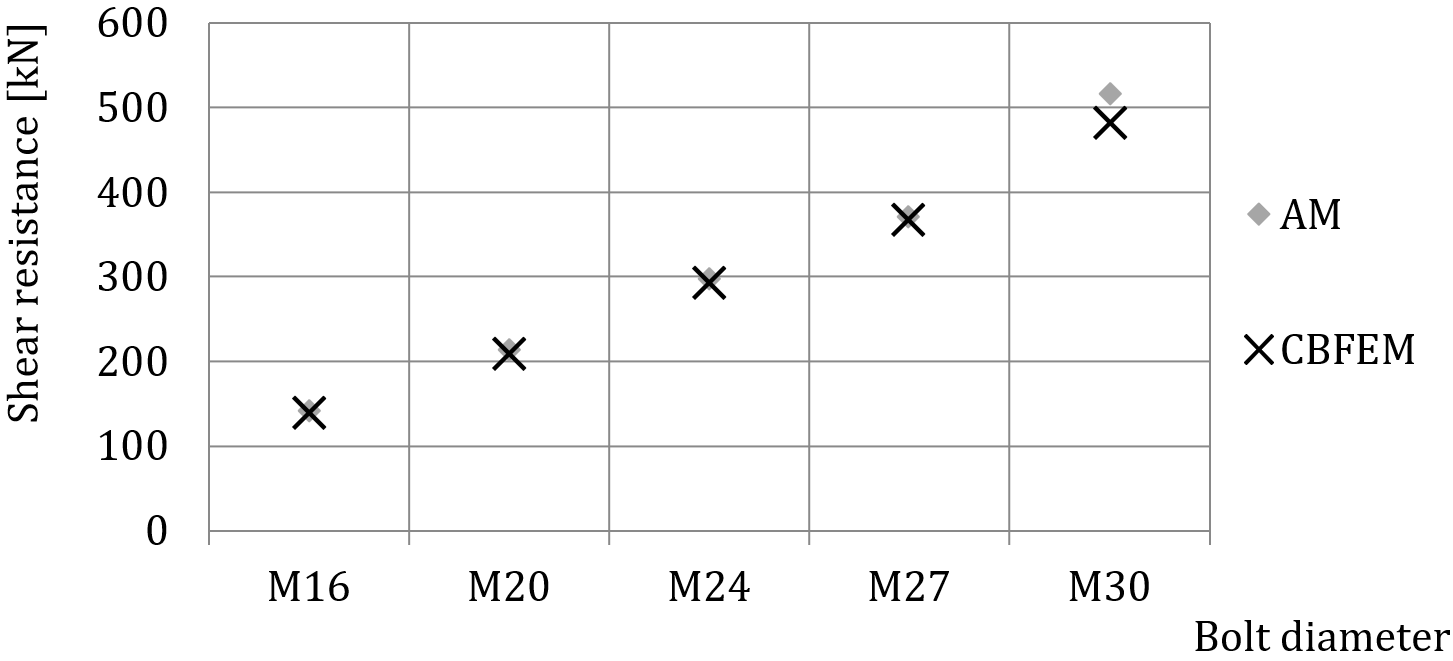Fig. 5.5.4 Sensitivity study for resistance in shear with variation of bolt diameter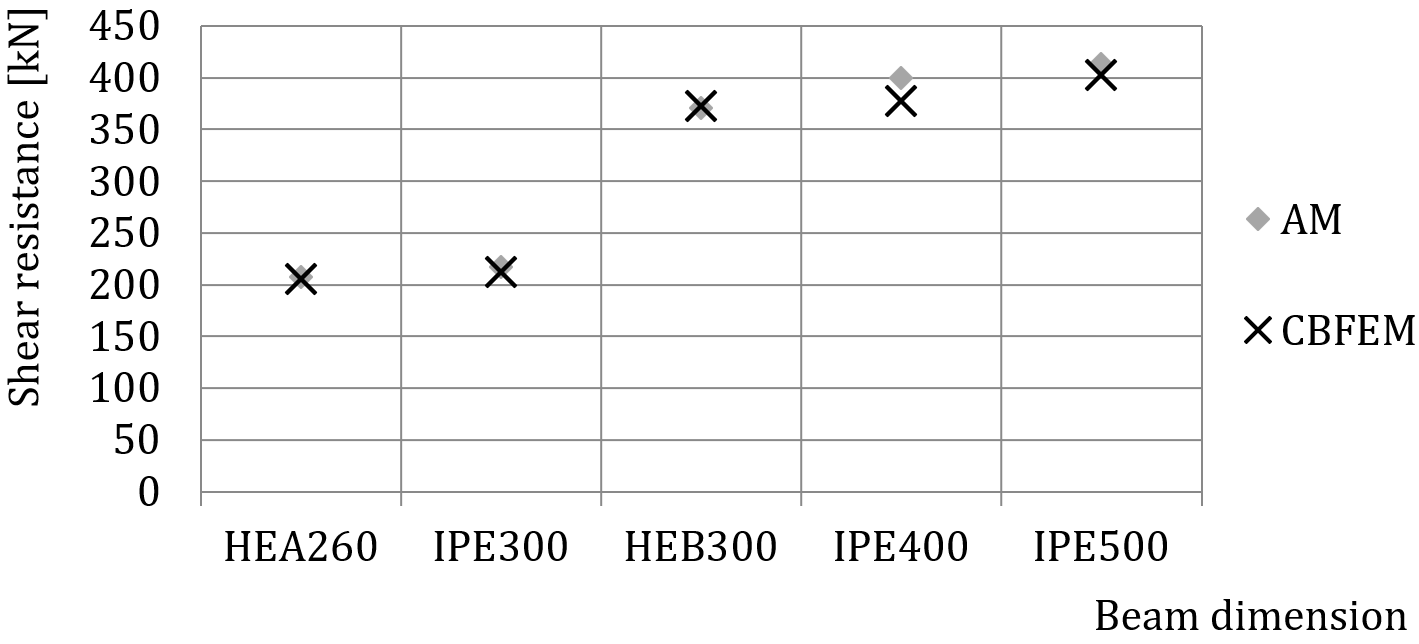Fig. 5.5.5 Sensitivity study for resistance in shear with variation of beam dimensionTab. 5.5.1 Sensitivity study for resistance with variation of bolt diameter Parameter AM CBFEM AM/CBFEM Beam; end plateDiameterDistancesMRd [kNm]VRd [kN]MRd [kNm]VRd [kN]MRd VRd IPE270; tp = 30mm; 150×310mmM16/8.8e1 = 60 mm; p1 = 190 mm; w1 = 30mm; w = 90 mm42142411401,021,02 M20/8.8e1 = 70 mm; p1 = 170 mm; w1 = 50 mm; w = 90 mm63213622091,021,02 HEA300; tp = 40mm; 300×330mmM24/8.8e1 = 85 mm; p1 = 160 mm; w1 = 75 mm; w = 150 mm95297942941,011,01 M27/8.8e1 = 95 mm; p1 = 140 mm; w1 = 75 mm; w = 150 mm1223711213681,011,01 M30/8.8e1 = 95 mm; p1 = 140 mm; w1 = 75 mm; w = 150 mm1455161394821,071,07 Tab. 5.5.2 Sensitivity study for resistance with variation of the beam dimension ParameterAMAMCBFEMCBFEMAM/CBFEMAM/CBFEM Beam; fin plateDiameterDistancesMRd [kNm]VRd [kN]MRd [kNm]VRd [kN]MRdVRd HEA260; tp = 25mm; 260×290mmM20/8.8e1 = 75 mm; p1 = 140 mm; w1 = 65 mm; w = 130 mm57207562051,011,01 IPE300; tp = 30mm; 150×340mmM20/8.8e1 = 70 mm; p1 = 200 mm; w1 = 35 mm; w = 90 mm71217692121,021,02 HEB300; tp = 40mm; 300×340mmM27/8.8e1 = 100 mm; p1 = 140 mm; w1 = 75 mm; w = 150 mm1223711223731,001,00 IPE400; tp = 45mm; 210×460mmM27/8.8e1 = 105 mm; p1 = 250 mm; w1 = 40 mm; w = 130 mm1763991673781,061,06 IPE500; tp = 45mm; 220×560mmM27/8.8e1 = 105 mm; p1 = 350 mm; w1 = 40 mm; w = 120 mm2264132214031,021,02 The results of sensitivity studies are summarized in graphs in Fig. 5.5.6 and 5.5.7. The results show that the differences between the two calculation methods are below 10 %. The analytical model gives generally higher resistance.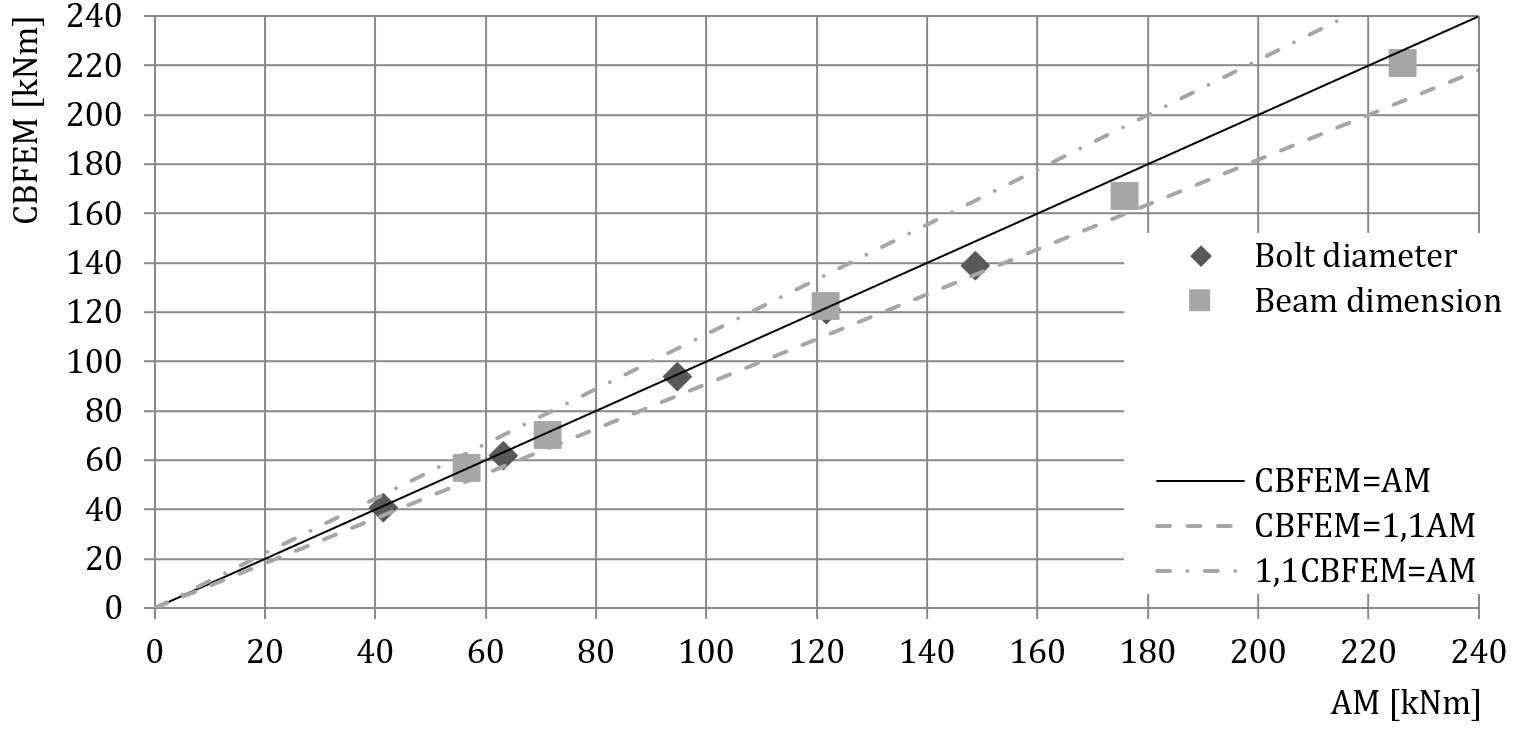Fig. 5.5.6 Verification of CBFEM to AM for the interaction of shear and tension in bolt in case of loading to bending resistance of a joint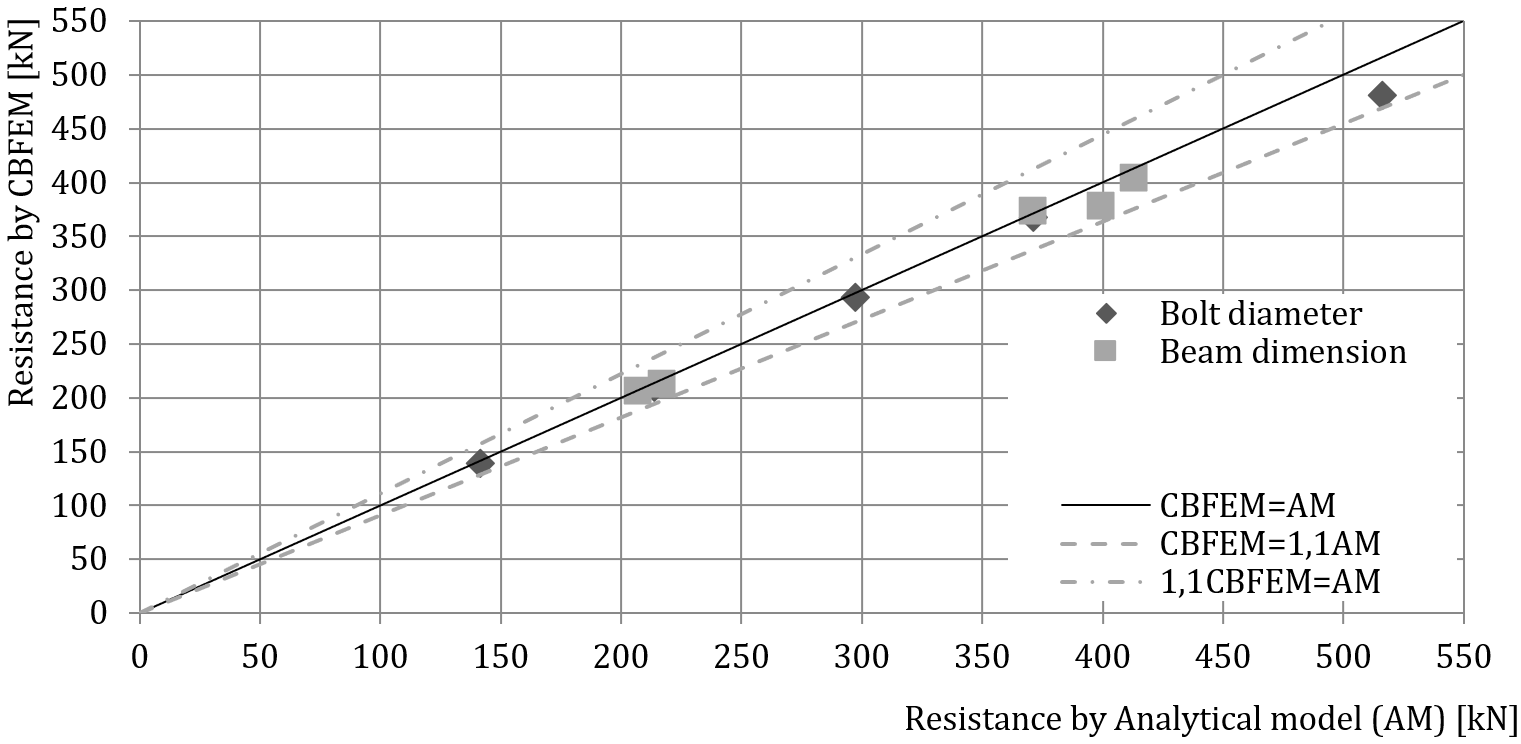Fig. 5.5.7 Verification of CBFEM to AM for the interaction of shear and tension in bolt in case of loading to shear resistance of a jointBenchmark exampleInputsConnected members Steel S355 Beams HEA300 End plate thickness tp = 40 mm End plate dimensions 300 × 330 mm Bolts 4 × M24 8.8 Distances e1 = 85 mm; p1 = 160 mm; w1 = 75 mm; w = 150 mm Outputs Design resistance in bending MRd = 94 kNm Design resistance in shear VRd = 294 kNm Collapse mode is bolt failure in interaction of shear and tension Sample files Open in Viewer Download$$